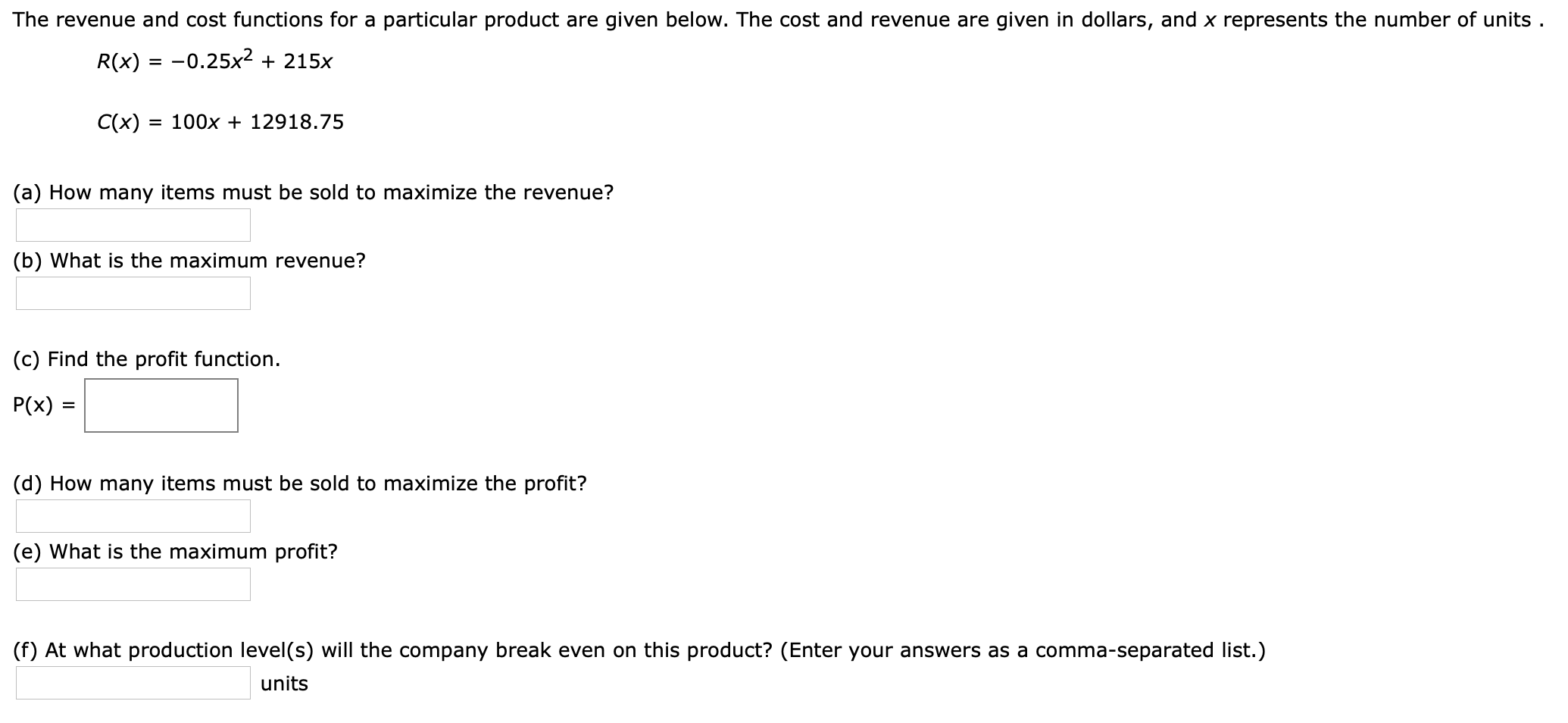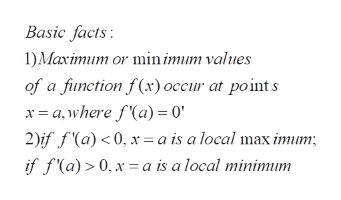# The revenue and cost functions for a particular product are given below. The cost and revenue are given in dollars, and x represents the number of units .R(x) = -0.25x2 215xC(x) = 100x + 12918.75(a) How many items must be sold to maximize the revenue?(b) What is the maximum revenue?(c) Find the profit functionP(x)(d) How many items must be sold to maximize the profit?(e) What is the maximum profit?(f) At what production level(s) will the company break even on this product? (Enter your answers as a comma-separated list.)units

Questionhelp_outlineImage TranscriptioncloseThe revenue and cost functions for a particular product are given below. The cost and revenue are given in dollars, and x represents the number of units . R(x) = -0.25x2 215x C(x) = 100x + 12918.75 (a) How many items must be sold to maximize the revenue? (b) What is the maximum revenue? (c) Find the profit function P(x) (d) How many items must be sold to maximize the profit? (e) What is the maximum profit? (f) At what production level(s) will the company break even on this product? (Enter your answers as a comma-separated list.) units fullscreen
check_circle

Step 1

As per norms, the first three questions are answered. The problem concerns finding optimium (extreme) values of the given functions

Step 2

How to determine the local maxima and minima o...help_outlineImage TranscriptioncloseBasic facts 1)Maximum or min imum values of a function f(x) occur at points a,where f(a)= 0 2)if f(a)<0, x= a is a local max imum if f(a) 0, x= a is a local minimum fullscreen

### Want to see the full answer?

See Solution

#### Want to see this answer and more?

Solutions are written by subject experts who are available 24/7. Questions are typically answered within 1 hour.*

See Solution
*Response times may vary by subject and question.
Tagged in

### Math